## 《Python之BMI计算》

《Python之BMI计算》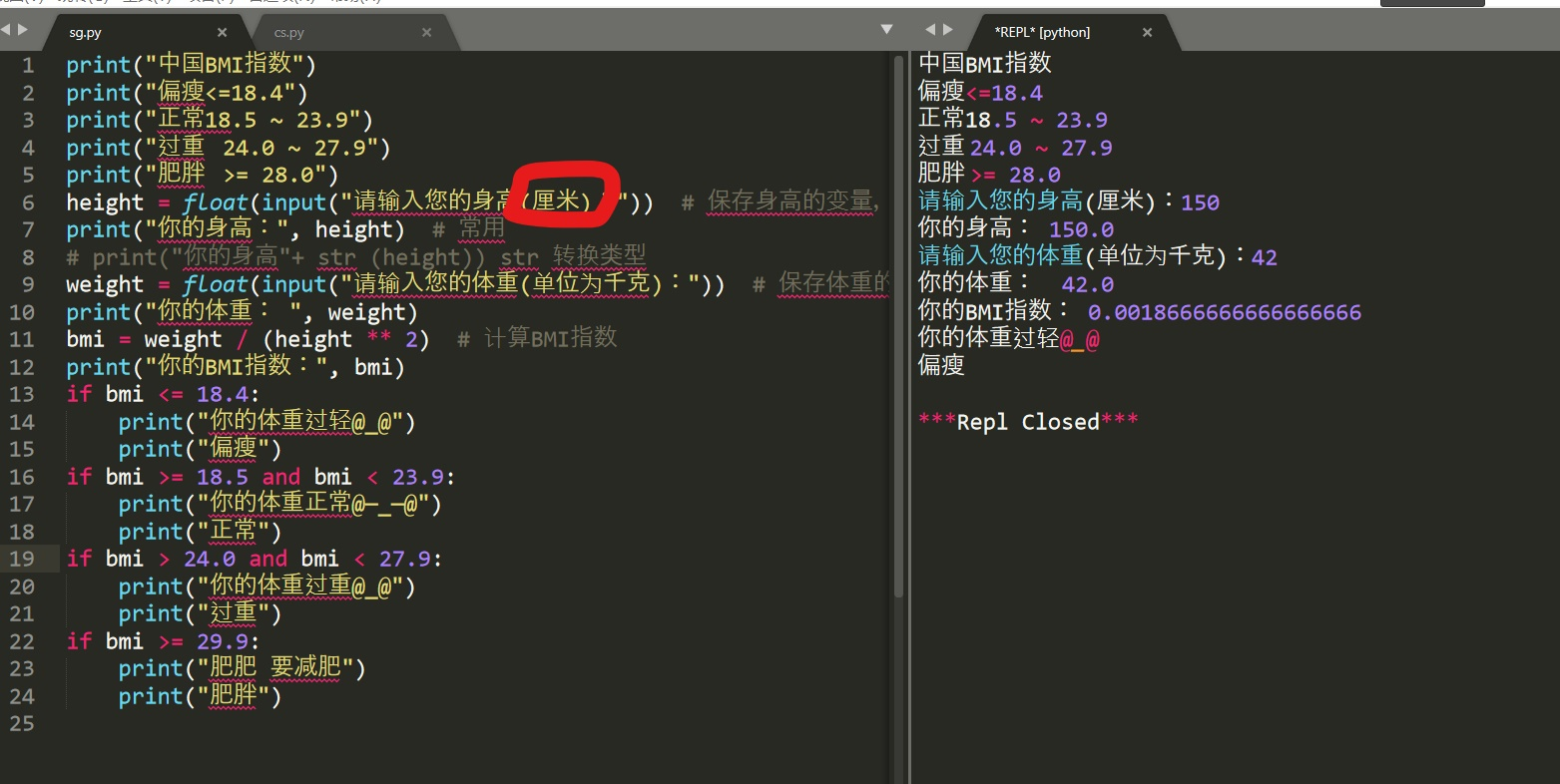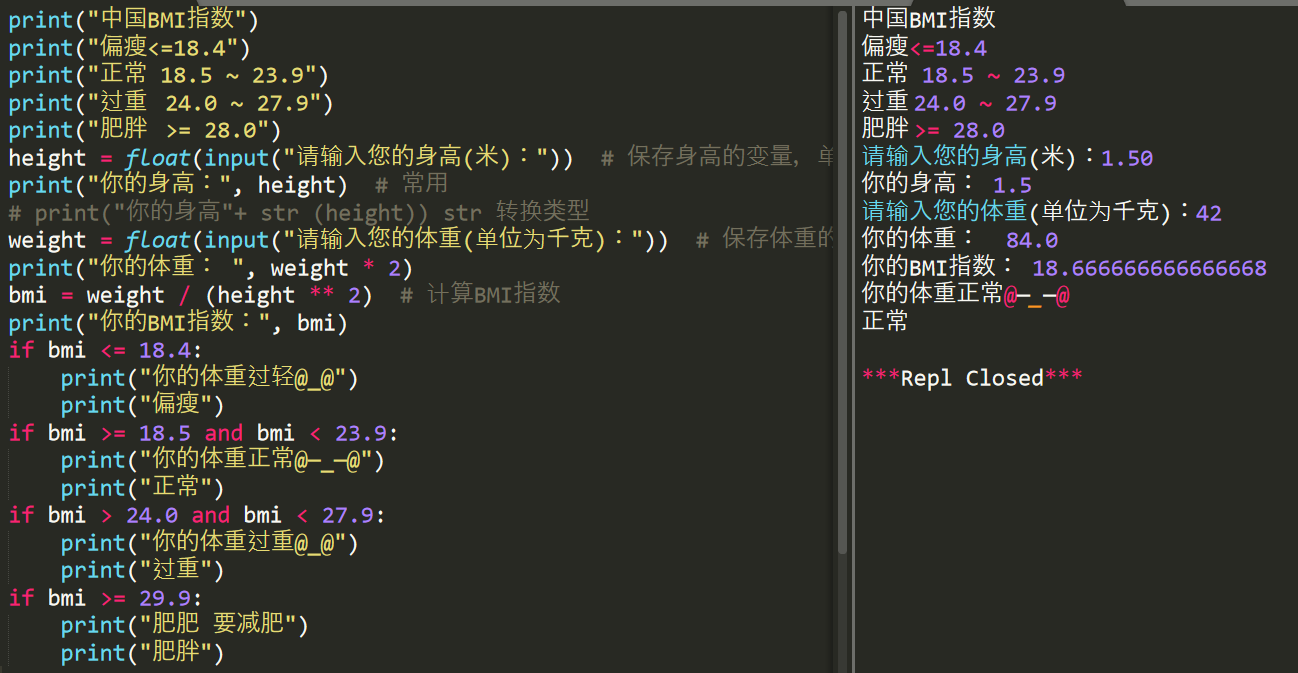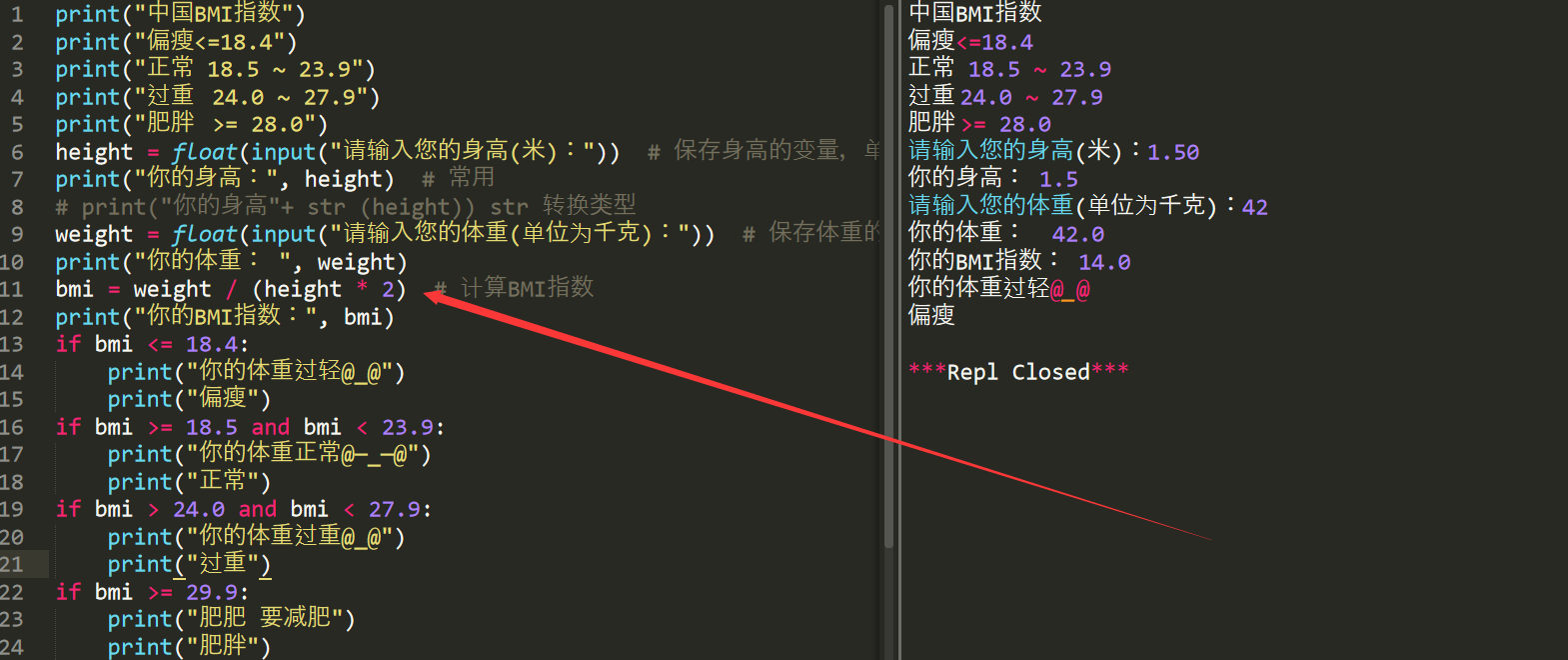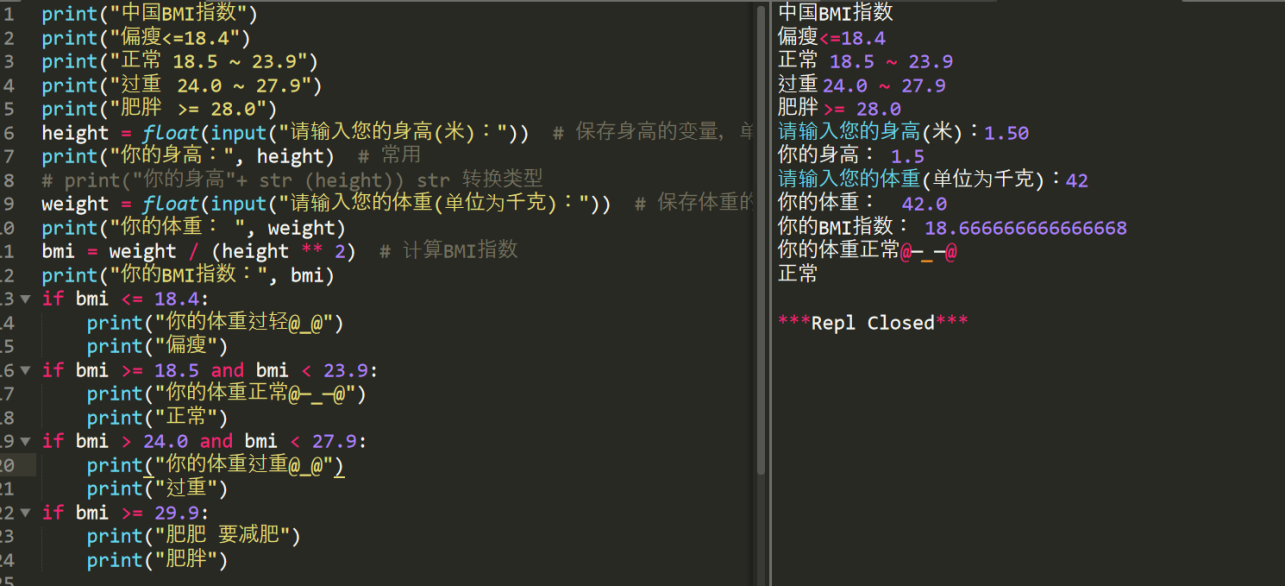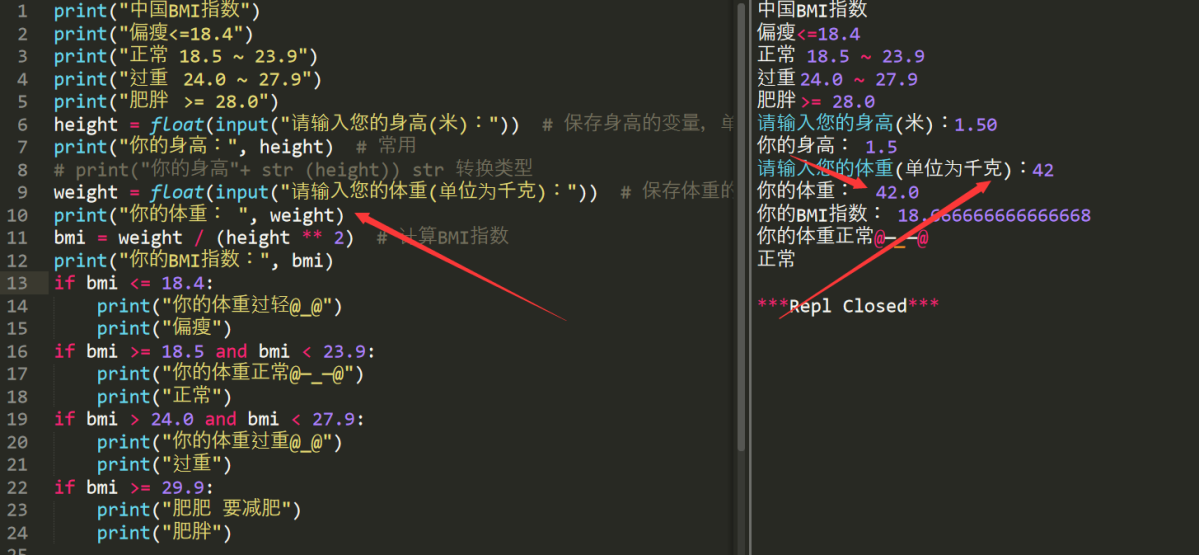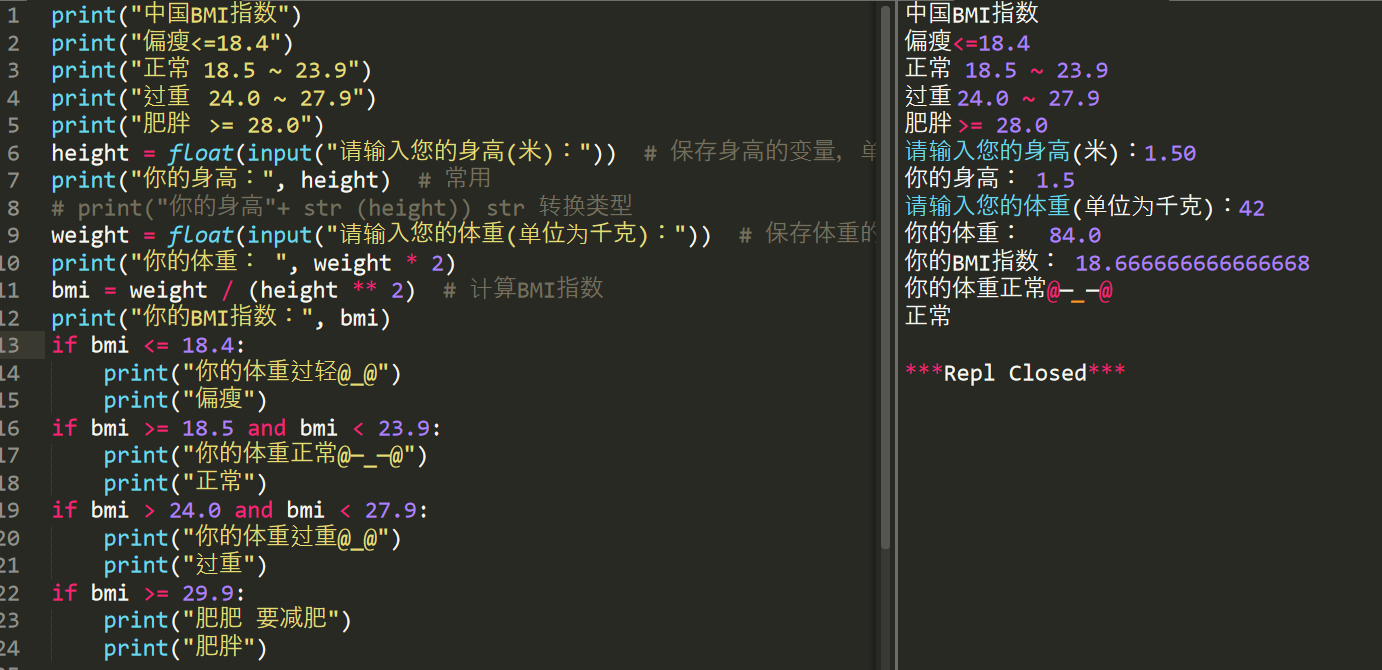print("中国BMI指数")

print("偏瘦<=18.4")

print("正常 18.5 ~ 23.9")

print("过重   24.0 ~ 27.9")

print("肥胖   >= 28.0")

height = float(input("请输入您的身高(米)："))  # 保存身高的变量，单位：米

print("你的身高：", height)  # 常用

# print("你的身高"+ str (height)) str 转换类型

weight = float(input("请输入您的体重(单位为千克)："))  # 保存体重的变量，单位：千克

print("你的体重： ", weight * 2)

bmi = weight / (height ** 2)  # 计算BMI指数

print("你的BMI指数：", bmi)

if bmi <= 18.4:

print("你的体重过轻@[email protected]")

print("偏瘦")

if bmi >= 18.5 and bmi < 23.9:

print("你的体重正常@—_—@")

print("正常")

if bmi > 24.0 and bmi < 27.9:

print("你的体重过重@[email protected]")

print("过重")

if bmi >= 29.9:

print("肥肥 要减肥")

print("肥胖")